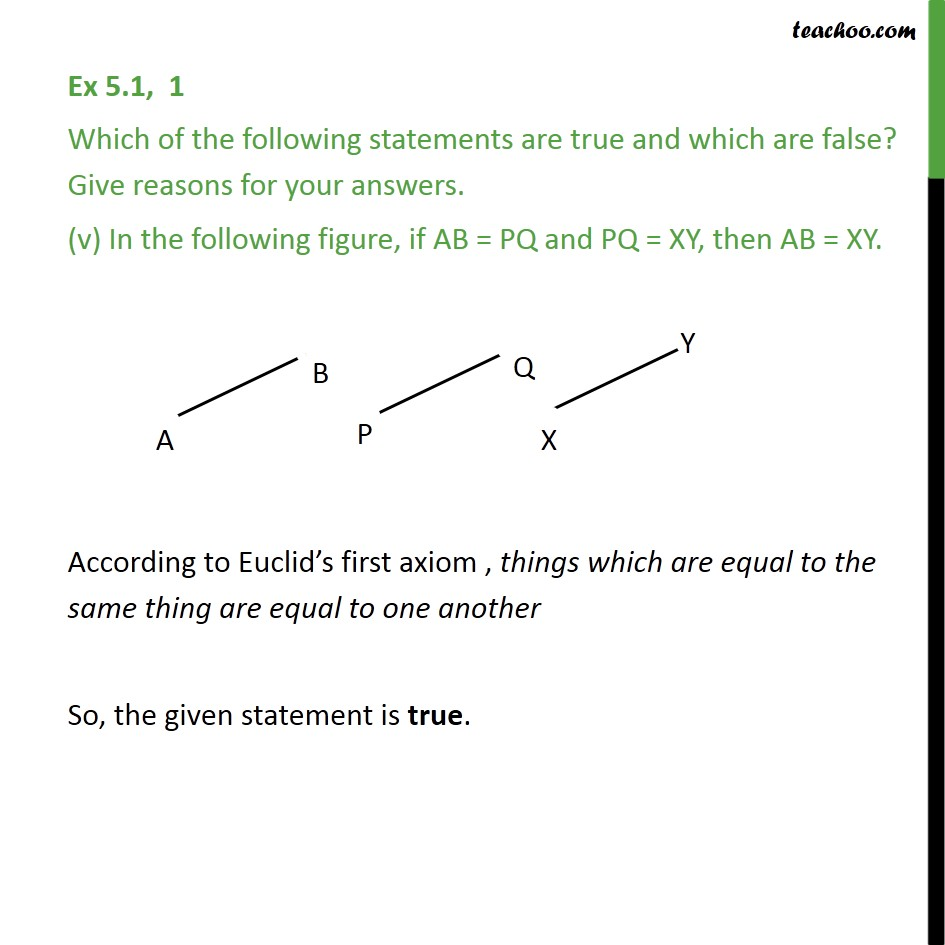Ex 5.1

Chapter 5 Class 9 Introduction to Euclid's Geometry
Serial order wiseLearn in your speed, with individual attention - Teachoo Maths 1-on-1 Class

### Transcript

Ex 5.1, 1 Which of the following statements are true and which are false? Give reasons for your answers. (v) In the following figure, if AB = PQ and PQ = XY, then AB = XY. According to Euclid’s first axiom , things which are equal to the same thing are equal to one another So, the given statement is true.